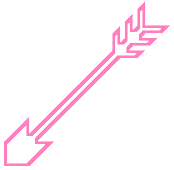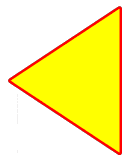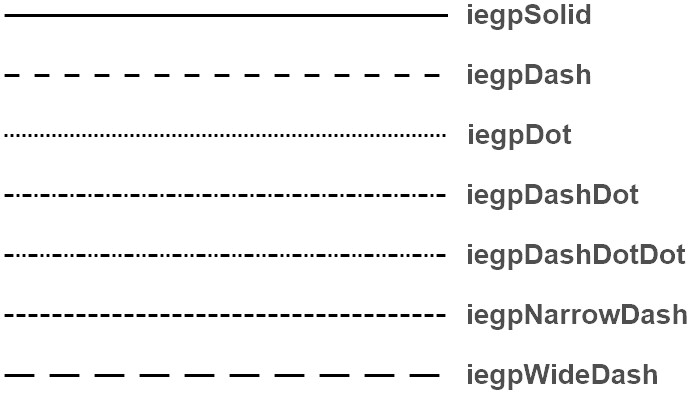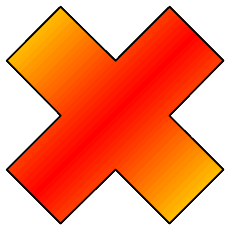ImageEn, unit iegdiplus

#### Declaration

PolylineClosed: boolean;
// Line Props
LineColor: TColor = clBlack;
LineWidth: Integer = 1;
LineStyle: TIEGPPenStyle;
LineOpacity: Double = 1.0;
// Fill Props
FillColor: TColor = clWhite;
FillColor2: TColor = clBlue;
FillOpacity: Double = 1.0;
// Other
Angle: Integer = 0;
AspectRatio: Double = 0.0;
AntiAlias: Boolean = True;
CalculateOnly: Boolean = False
): TSize;

#### Description

Draw a polyline or polygon.For a simpler method, use: Polyline or Polygon.

General Properties
Parameter Description
Points The list of the points that make up the polyline
PolylineClosed Set to true to make a polygon (a filled polyline)
LineColor The color of the line (or clNone for no border)
LineWidth The width of the line
LineStyle How the line is drawn
LineOpacity Specifies the opacity of the line (from 0.0 to 1.0)
FillColor The color of the polygon or clNone for no fill (PolylineClosed must be true)
FillColor2 The color of the secondary fill (if the polygon fill has a gradient)
FillGradient The direction of the gradient for the polygon fill (from FillColor to FillColor2). gpgNone specifies no gradient
FillOpacity Specifies the opacity of the polygon fill (from 0.0 to 1.0)

Other Parameters
 Angle Optionally specify any rotation of the drawing (in degrees counter-clockwise, i.e. 90 rotates left) AspectRatio Optionally specify any locking of the drawing, e.g. 1.0 would enforce 1:1 sizing. 0 means no locking AntiAlias True uses best quality drawing. False disables anti-aliasing CalculateOnly If true, the text is not drawn, just the output size returned as the result

Result is the area of the entire draw in pixels.#### Example

// Draw a cross
var
pts: array of TPoint;
begin
SetLength( pts, 12 );
pts := Point( 50, 0 );
pts := Point( 100, 50 );
pts := Point( 150, 0 );
pts := Point( 200, 50 );
pts := Point( 150, 100 );
pts := Point( 200, 150 );
pts := Point( 150, 200 );
pts := Point( 100, 150 );
pts := Point( 50, 200 );
pts := Point( 0, 150 );
pts := Point( 50, 100 );
pts := Point( 0, 50 );

clBlack, 2, 1.0,                       // Line Props
clYellow, clRed, gpgDiagCenter, 1.0 ); // Fill Props
ImageEnView1.Update();
end;